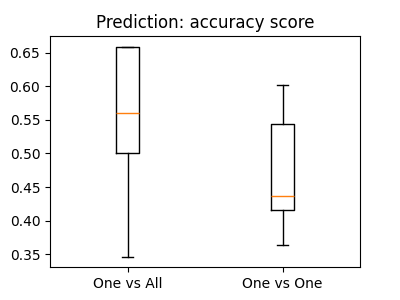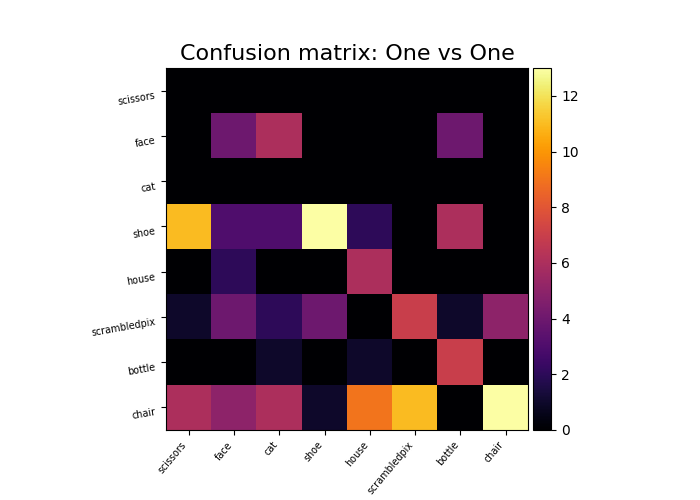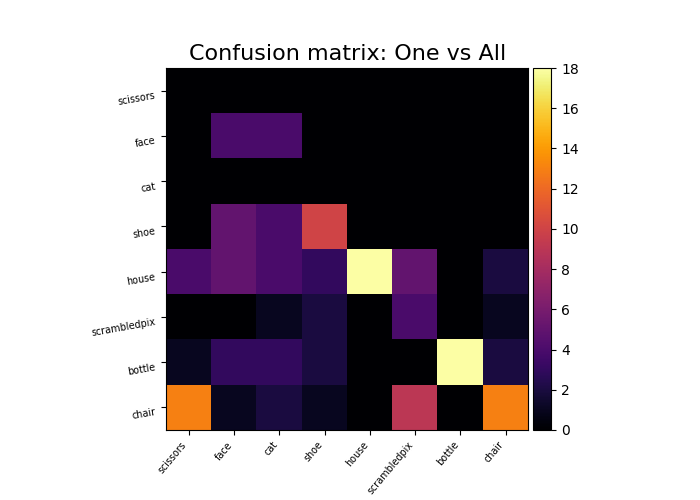# The haxby dataset: different multi-class strategies#

We compare one vs all and one vs one multi-class strategies: the overall cross-validated accuracy and the confusion matrix.

Note

If you are using Nilearn with a version older than `0.9.0`, then you should either upgrade your version or import maskers from the `input_data` module instead of the `maskers` module.

That is, you should manually replace in the following example all occurrences of:

```from nilearn.maskers import NiftiMasker
```

with:

```from nilearn.input_data import NiftiMasker
```

## Load the Haxby data dataset#

```import numpy as np
import pandas as pd

from nilearn import datasets

# By default 2nd subject from haxby datasets will be fetched.
haxby_dataset = datasets.fetch_haxby()

# Print basic information on the dataset
print(f"Functional nifti images are located at: {haxby_dataset.func}")

func_filename = haxby_dataset.func

# Load the behavioral data that we will predict
y = labels["labels"]
session = labels["chunks"]

# Remove the rest condition, it is not very interesting
non_rest = y != "rest"
y = y[non_rest]

# Get the labels of the numerical conditions represented by the vector y
unique_conditions, order = np.unique(y, return_index=True)
# Sort the conditions by the order of appearance
unique_conditions = unique_conditions[np.argsort(order)]
```
```Mask nifti images are located at: /home/runner/work/nilearn/nilearn/nilearn_data/haxby2001/mask.nii.gz
Functional nifti images are located at: /home/runner/work/nilearn/nilearn/nilearn_data/haxby2001/subj2/bold.nii.gz
```

## Prepare the fMRI data#

```from nilearn.maskers import NiftiMasker

# For decoding, standardizing is often very important
standardize="zscore_sample",
runs=session,
smoothing_fwhm=4,
memory="nilearn_cache",
memory_level=1,
)

# Remove the "rest" condition
X = X[non_rest]
session = session[non_rest]
```
```/usr/share/miniconda3/envs/testenv/lib/python3.9/site-packages/nilearn/image/resampling.py:493: UserWarning:

The provided image has no sform in its header. Please check the provided file. Results may not be as expected.
```

## Build the decoders, using scikit-learn#

Here we use a Support Vector Classification, with a linear kernel, and a simple feature selection step

```from sklearn.feature_selection import SelectKBest, f_classif
from sklearn.multiclass import OneVsOneClassifier, OneVsRestClassifier
from sklearn.pipeline import Pipeline
from sklearn.svm import SVC

svc_ovo = OneVsOneClassifier(
Pipeline(
[
("anova", SelectKBest(f_classif, k=500)),
("svc", SVC(kernel="linear")),
]
)
)

svc_ova = OneVsRestClassifier(
Pipeline(
[
("anova", SelectKBest(f_classif, k=500)),
("svc", SVC(kernel="linear")),
]
)
)
```

## Now we compute cross-validation scores#

```from sklearn.model_selection import cross_val_score

cv_scores_ovo = cross_val_score(svc_ovo, X, y, cv=5, verbose=1)

cv_scores_ova = cross_val_score(svc_ova, X, y, cv=5, verbose=1)

print("OvO:", cv_scores_ovo.mean())
print("OvA:", cv_scores_ova.mean())
```
```OvO: 0.601855088049469
OvA: 0.6712058072321548
```

## Plot barplots of the prediction scores#

```from matplotlib import pyplot as plt

plt.figure(figsize=(4, 3))
plt.boxplot([cv_scores_ova, cv_scores_ovo])
plt.xticks([1, 2], ["One vs All", "One vs One"])
plt.title("Prediction: accuracy score")
``````Text(0.5, 1.0, 'Prediction: accuracy score')
```

## Plot a confusion matrix#

We fit on the first 10 sessions and plot a confusion matrix on the last 2 sessions

```from sklearn.metrics import confusion_matrix

from nilearn.plotting import plot_matrix, show

svc_ovo.fit(X[session < 10], y[session < 10])
y_pred_ovo = svc_ovo.predict(X[session >= 10])

plot_matrix(
confusion_matrix(y_pred_ovo, y[session >= 10]),
labels=unique_conditions,
title="Confusion matrix: One vs One",
cmap="hot_r",
)

svc_ova.fit(X[session < 10], y[session < 10])
y_pred_ova = svc_ova.predict(X[session >= 10])

plot_matrix(
confusion_matrix(y_pred_ova, y[session >= 10]),
labels=unique_conditions,
title="Confusion matrix: One vs All",
cmap="hot_r",
)

show()
```
••Total running time of the script: (0 minutes 57.289 seconds)

Estimated memory usage: 3129 MB

Gallery generated by Sphinx-Gallery